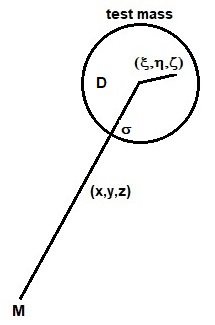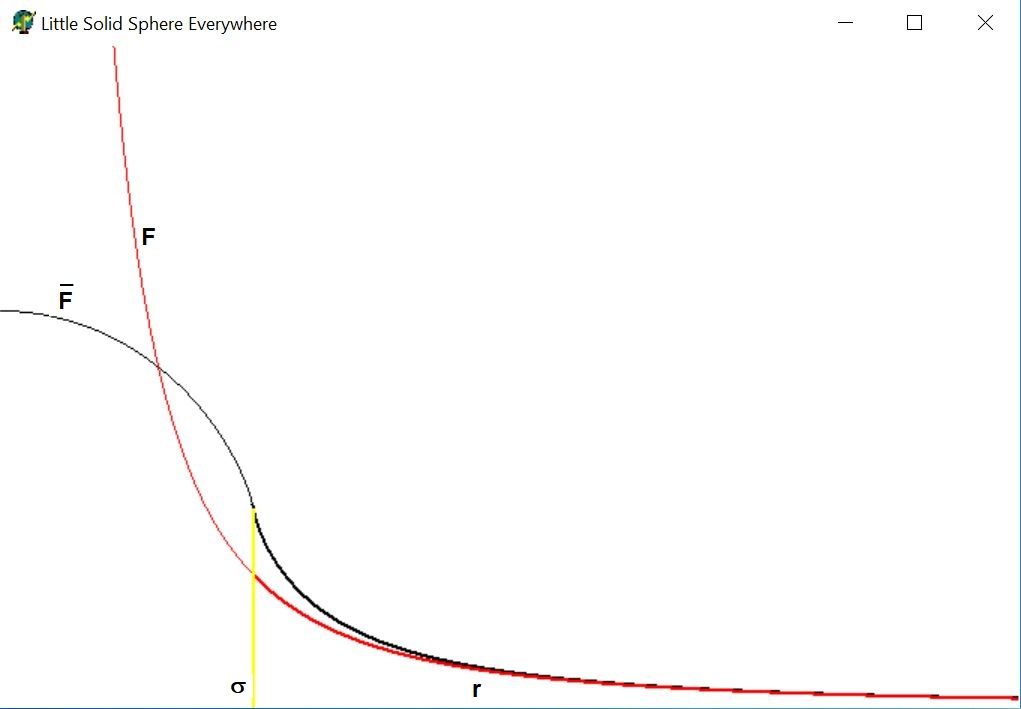# Sensible Gravity

### Author: Han de Bruijn Date: 2018 November

Relevant reference: Shell theoremMeasuring a force of gravity can be modeled with a spherical test mass hanging on a string, where the stretching of the string is a measure for the strength of the force. Mathematically , the finite size of the test mass results in sort of a convolution integral with a rectangular distribution in 3-D as its kernel. The solid sphere approximation makes an analytical ("exact") treatment possible. Since basically everything in physics is subject to experimental observation, the very nature of any function in physics is revealed by such a convolution integral. The aim of this paper is to demonstrate that a singularity of the form $1/r^{2}$ in three-dimensional space, such as with the gravity field of an point mass, is actually non-existent in this sense. So let's investigate what sensible gravity is.

Let $\sigma$ be the radius of the little solid sphere, $(\xi,\eta,\zeta)$ its local (Cartesian) coordinates and $\rho,\theta,\phi$ the spherical coordinates equivalent of the latter. Then the test mass is modeled as: $$S(\xi,\eta,\zeta) = \left\{ \begin{array}{ll} D & \mbox{for} \quad \xi^2+\eta^2+\zeta^2 < \sigma^2 \\ 0 & \mbox{for} \quad \xi^2+\eta^2+\zeta^2 > \sigma^2 \end{array} \right. \quad \mbox{or} \quad S(\rho) = \left\{ \begin{array}{ll} D & \mbox{for} \quad \rho < \sigma \\ 0 & \mbox{for} \quad \rho > \sigma \end{array} \right.$$ Where $D$ is the (uniform) density of the solid sphere, which can be expressed in its mass $m$ and its size $\sigma$: $$D \iiint S(\xi,\eta,\zeta) \, d\xi\, d\eta\, d\zeta = D \frac{4}{3}\pi \sigma^3 = m \quad \Longleftrightarrow \quad D = m / \left(\frac{4}{3}\pi \sigma^3\right)$$ The gravity field $F$ of the point mass $M$ to be measured is given by: $$F(r) = G\frac{m\cdot M}{r^2} = G\frac{m\cdot M}{x^2 + y^2 + z^2}$$ Where $m$ is the mass of the solid test sphere, $M$ is the point mass causing the gravitational force to be measured, $G$ is the gravitational constant. Now let $\vec{r}=(x,y,z)$ be the vector pointing to the center of the solid test sphere with our point mass $M$ as the origin. The force at the test mass as a whole is then calculated as follows: $$\overline{F}(x,y,z) = G\cdot M\cdot \iiint S(\xi,\eta,\zeta) \frac{D\,d\xi\, d\eta\, d\zeta}{(x+\xi)^2 + (y+\eta)^2 + (z+\zeta)^2}$$ Introducing spherical coordinates: $$\left\{ \begin{array}{l} \xi = \rho \sin(\theta) \cos(\phi) \\ \eta = \rho \sin(\theta) \sin(\phi) \\ \zeta = \rho \cos(\theta) \end{array} \right. \qquad \mbox{where} \quad 0 \le \theta \le \pi \quad \mbox{and} \quad 0 \le \phi \le 2\pi$$ Because of the expected spherical symmetry of the problem, we only consider a ray in $z$ direction, which means that $x = 0$ , $y = 0$ and $z = r$ . The integral then becomes, after some suitable rearrangement: $$\overline{F}(r) = G\cdot M\cdot m / \left(\frac{4}{3}\pi \sigma^3\right) \int_0^{2\pi} d\phi \int_0^\sigma d\rho \int_0^\pi \frac{\rho^2 \sin(\theta) \, d\theta} {\rho^2 \sin^2(\theta) + \left[r + \rho \cos(\theta)\right]^2}$$ Let's proceed with an abbreviation for the constant factor: $$C = G\cdot M\cdot m / \left(\frac{4}{3}\pi \sigma^3\right)$$ Then: $$\overline{F}(r) = -2\pi\,C \int_0^\sigma d\rho \int_0^\pi \frac{\rho^2 \left[-\sin(\theta)\right] \, d\theta} {\rho^2 + r^2 + 2\, r\rho \cos(\theta)}$$ $$= -2\pi\,C \int_0^\sigma d\rho \frac{\rho}{2 r} \int_{\theta=0}^{\theta=\pi} \frac{d\left[\rho^2 + r^2 + 2\, r\rho\cos(\theta)\right]} {\rho^2 + r^2 + 2\, r\rho \cos(\theta)}$$ $$= -C\, \frac{2\pi}{2\, r} \int_0^\sigma \rho \, d\rho \left[ \ln\left(\rho^2 + r^2 + 2\, r\rho\cos(\theta)\right) \right]_{\theta=0}^{\theta=\pi}$$ $$= + C\, \frac{2\pi}{2\, r} \int_0^\sigma \left\{ \ln\left[(\rho + r)^2\right] - \ln\left[(\rho - r)^2\right] \right\} \rho \, d\rho$$ Substitution of $x = \rho/\sigma$ and $s = r/\sigma$ gives: $$\overline{F}(r) = C\, \frac{\pi\,\sigma^2}{r} \, \int_0^1 \left\{ \ln\left[(x + s)^2\right] - \ln\left[(x - s)^2\right] \right\} x \, dx$$ Divide and conquer one: $$\int \ln(x+s)^2 x \, dx =$$ $$\int \ln(x+s)^2 (x+s) \, d(x+s) - s \int 2\, \ln|x+s| \, d(x+s) =$$ $$\frac{1}{2} (x+s)^2 \ln(x+s)^2 - \frac{1}{2}(x+s)^2 - 2 \, s \left[ (x+s)\ln|x+s| - (x+s) \right] =$$ $$(x^2 + 2 x s + s^2) \ln|x+s| - x^2/2 - x s - s^2/2$$ $$+ (- 2 x s - 2 s^2) \ln|x+s| + 2 x s + 2 s^2 =$$ $$(x^2 - s^2) \ln|x+s| - x^2/2 + x s + 3 s^2/2$$ Divide and conquer two: $$\int \ln(x-s)^2 x \, dx =$$ $$\int \ln(x-s)^2 (x-s) \, d(x-s) + s \int 2\, \ln|x-s| \, d(x-s) =$$ $$\frac{1}{2} (x-s)^2 \ln(x-s)^2 - \frac{1}{2} (x-s)^2 + 2 \, s \left[ (x-s)\ln|x-s| - (x-s) \right] =$$ $$(x^2 - 2 x s + s^2) \ln|x-s| - x^2/2 + x s - s^2/2$$ $$+ (2 x s - 2 s^2) \ln|x-s| - 2 x s + 2 s^2 =$$ $$(x^2 - s^2) \ln|x-s| - x^2/2 - x s + 3 s^2/2$$ One and two must be substracted. And integrated from $0$ to $1$. $$\int_0^1 \left[ \ln(x+s)^2 - \ln(x-s)^2 \right] x \, dx =$$ $$\left[ (x^2 - s^2) \ln|x+s| - x^2/2 + x s + 3 s^2/2 \right]_{x=0}^{x=1} -$$ $$\left[ (x^2 - s^2) \ln|x-s| - x^2/2 - x s + 3 s^2/2 \right]_{x=0}^{x=1} =$$ $$\left[ (x^2 - s^2) \ln \left| \frac{x+s}{x-s} \right| + 2\, x s \right]_{x=0}^{x=1} = (1 - s^2) \ln \left| \frac{1+s}{1-s} \right| + 2\, s$$ Consequently, the gravitational field as felt by the test mass is: $$\overline{F}(r) = C\, \frac{\pi\,\sigma^2}{r} \, \left\{ \left[1 - \left(\frac{r}{\sigma}\right)^2\right] \ln \left| \frac{1+r/\sigma}{1-r/\sigma} \right| + 2\, \frac{r}{\sigma} \right\}$$ There are a few singularities involved here. The first one is at $r=0$ , which is easiest to calculate if we start from the basics all over. $$\overline{F}(0,0,0) = G\cdot M \cdot \iiint S(\xi,\eta,\zeta) \frac{d\xi\, d\eta\, d\zeta}{\xi^2 + \eta^2 + \zeta^2} \quad \Longleftrightarrow \quad$$ $$\overline{F}(0) = G\cdot M \cdot m / \left(\frac{4}{3}\pi \sigma^3\right) \int_0^\sigma \frac{4\pi\rho^2\, d\rho}{\rho^2} = C\, 4\pi\, \sigma = \frac{3\,G M m}{\sigma^2}$$ The next singularity is where the denominator $(1-r/\sigma)$ in the logarithm for $r=\sigma$ , or $(1-s)$ for $s=1$ , becomes zero. $$\lim_{s\rightarrow 1} \left\{ (1 - s^2) \ln \left| \frac{1+s}{1-s} \right| + 2\, s \right\} =$$ $$\lim_{s\rightarrow 1} \left\{ (1+s)(1-s)\ln|1+s| - (1+s)\left[ (1-s)\ln|1-s| \right] + 2\, s \right\} = 2$$ Because $\lim_{x\rightarrow 0} x\ln(x) = 0$ with $x = (1-s)$ . $$\quad \Longrightarrow \quad \overline{F}(\sigma) = C\, \frac{\pi\,\sigma^2}{\sigma}\, 2 = C\, 2\pi\, \sigma = \frac{1}{2} \overline{F}(0) = \frac{3\,G M m}{2\,\sigma^2} = \frac{3}{2} F(\sigma)$$ We could have done it by hand, but with MAPLE it goes faster:

> simplify(diff((1-s^2)*ln((1+s)/(1-s))+2*s,s));

$$-2 s \ln\left|\frac{1+s}{1-s}\right| + 4$$ From this we see that for $s=1$ i.e. $r=\sigma$ the function $\overline{F}(r)$ has a slope that is negative and infinitely large: $\overline{F'}(\sigma) = -\infty$ .
The force of gravity as felt by the test mass can also be written as follows: $$\overline{F}(r) = C\, \frac{\pi\,\sigma^2}{r} \frac{r^2}{\sigma^2} \left\{ \left[\left(\frac{\sigma}{r}\right)^2 - 1\right] \ln \left| \frac{\sigma/r+1}{\sigma/r-1} \right| + 2\, \frac{\sigma}{r} \right\}$$ And with help of the variable $x = \sigma/r$ , which is small for $r \gg \sigma$ : $$= \frac{3\, G M m }{4\, \sigma^2 x} \left\{ (x^2 - 1) \ln\left|\frac{x+1}{x-1}\right| + 2\, x \right\}$$ We could have done it by hand, but with MAPLE it goes much faster:
> series(3/4/(\sigma^2*x)*((x^2-1)*ln((1+x)/(1-x))+2*x),x,7);

$$\frac{1}{\sigma^2} x^2 + \frac{1}{5 \sigma^2} x^4 + \frac{3}{35 \sigma^2} x^6 + O(x^8) \approx \frac{1}{\sigma^2} \left( \frac{\sigma}{r} \right)^2 = \frac{1}{r^2}$$ Thus the standard gravitation law (red) is valid for small values of $x$ , that is for large values of $r$ , that is for $r \gg\sigma$ . The law as actually observed within this model is depicted in black; yellow line at position $r = \sigma$ . See picture:Remember that $r$ is the distance of the center of the test mass $m$ to the point mass $M$. Thus for $r < \sigma$ the point mass would be penetrating the test mass, which means that the singularity would be inside the test mass. Which is physically improbable, therefore we have drawn thinner lines in that region. This would mean that gravity, in the end, has only sense for $r > \sigma$: with a test mass that is barely touching the singularity for $r=\sigma$.

### Disclaimers

Anything free comes without referee :-(
My English may be better than your Dutch :-)